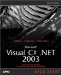The if Statement

The if statement selects a statement for execution based on the value of a Boolean expression. Here's what this statement looks like formally :

if (  expression  )  statement1  [else  statement2  ]

Here are the parts of this statement:

• expression An expression that can be implicitly converted to a bool value. If true , statement1 is executed, otherwise , statement2 (if present) will be executed.

• statement1 The statement(s) to be executed if expression is true . Can be a compound statement.

• statement2 The statement(s) to be executed if expression is false . Can be a compound statement.

You can see an example in ch01_10.cs, Listing 1.10, where we're playing Blackjack with a user. As long as the value the user enters beats or is equal to our value (18), but doesn't exceed 21, they win; otherwise, we win. To decide the outcome of the game, we use an if statement, as you can see in the code.

Listing 1.10 Using the if Statement (ch01_10.cs)
class ch01_10 {   static void Main()   {     int myNumber = 18;     System.Console.WriteLine("BlackJack!");     System.Console.Write("Can you beat my number? Enter 1-21: ");     int theirNumber = System.Convert.ToInt32(System.Console.ReadLine());     if (theirNumber >= myNumber && theirNumber <= 21)     {       System.Console.WriteLine("You win.");     } else {       System.Console.WriteLine("You lose.");     }   } }

Take a look at the if statement in this example:

if (theirNumber >= myNumber && theirNumber <= 21) {   System.Console.WriteLine("You win."); } else {   System.Console.WriteLine("You lose."); }

The expression that this if statement tests, theirNumber >= myNumber && theirNumber <= 21 , uses the relational operators >= (greater-than-or-equal-to) and <= ( less-than -or-equal-to) to create two logical clauses, theirNumber >= myNumber and theirNumber <= 21 . The logical operator && (the logical And operator) connects these clauses, insisting that the overall expression is true only if both these clauses are true. In this way, the number the user entered has to beat our number and be less than 21. If we had used the logical operator (the logical Or operator) instead, either of the two clauses could be true for the overall expression to be true.

Here's what you see when you run ch01_10:

C:\>ch01_10 BlackJack! Can you beat my number? Enter 1-21: 17 You lose. C:\>ch01_10 BlackJack! Can you beat my number? Enter 1-21: 20 You win.

A popular relational operator is the == operator, which tests for equality. For example, the expression variable == 5 is true if variable holds 5, false otherwise. In C++, confusing == and = in if statements is a classic mistakefor example, instead of writing if(temperature == 32)... ., one might write if(temperature = 32).... , and that's a problem because the second version assigns temperature a value of 32, no matter what its original value was. In addition, the expression temperature = 32 evaluates to 32, and because non-zero values evaluate to true in C++, the if statement's enclosed statement would be executed. C# solves this problem to a great extent by insisting that the conditional expressions evaluated by if statements must be of type bool , so if(temperature = 32)... wouldn't compile.

FOR C++ PROGRAMMERS

By demanding that the conditional expression in if statements be of a bool data type, C# goes a long way in eliminating the confusion between == and = .

Like C++, C# also makes its logical operators, such as && and , shortcut operators. If you use these operators to connect two logical clauses in an expression and the overall Boolean value of the expression becomes clear by evaluating the first clause, the second clause will not be evaluated ( short-circuiting the evaluation of the entire expression).

TESTING STRINGS FOR EQUALITY

Although string is a reference type, the equality operators ( == and != ) are defined to compare the values of string objects, not references.

For example, if in the expression theirNumber >= myNumber && theirNumber <= 21 , theirNumber is not greater than or equal to myNumber , the first clause is false , which means the Boolean value of the whole expression must be false . This in turn means that C# will not evaluate the second clause because it already knows the answer. That's something to remember in case the second clause does something vital you'll need later, like open a file.Microsoft Visual C#.NET 2003 Kick Start
ISBN: 0672325470
EAN: 2147483647
Year: 2002
Pages: 181

Similar book on Amazon